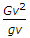# Civil Engineering - Applied Mechanics

### Exercise :: Applied Mechanics - Section 3

11.

Time of flight of a projectile on a horizontal plane, is

 A.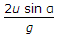B.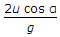C.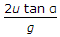D.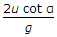Explanation:

No answer description available for this question. Let us discuss.

12.

The reaction RB of the roller support B of the beam shown in below figure is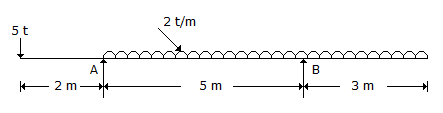A. 10.8 t B. 10.6 t C. 10.4 t D. 10.2 t.

Explanation:

No answer description available for this question. Let us discuss.

13.

The acceleration of a train starting from rest at any instant is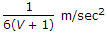where V is the velocity of the train in m/sec. The train will attain a velocity of 36 km/hour after travelling a distance of

 A. 2000 m B. 2100 m C. 2200 m D. 2300 m E. 2500 m.

Explanation:

No answer description available for this question. Let us discuss.

14.

The maximum frictional force which comes into play, when a body just begins to slide over the surface of a an other body, is known

 A. sliding friction B. rolling friction C. limiting friction D. none of these.

Explanation:

No answer description available for this question. Let us discuss.

15.

If G is the Gauge of track, v is velocity of the moving vehicle, g is the acceleration due to gravity and r is the radius of a circular path, the required superelevation is

 A.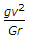B.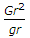C.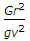D.